Courses

# Elastic Constants Notes | EduRev

## Mechanical Engineering : Elastic Constants Notes | EduRev

The document Elastic Constants Notes | EduRev is a part of the Mechanical Engineering Course Strength of Materials (SOM).
All you need of Mechanical Engineering at this link: Mechanical Engineering

Elastic constants

Elastic constants are those factors which determine the deformations produced by a given stress system acting on a material.

Various elastic constants are:

1. Modulus of elasticity (E)
2. Poisson’s ratio (μ or 1/m)
3. Modulus of rigidity (G or N)
4. Bulk modulus (K)

Materials on the basis of elastic properties

• Homogeneous Material
When a material exhibits Same elastic properties at any point in a given directions than the material is known as homogenous material.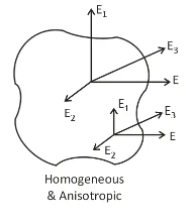Homogeneous material

• Isotropic Material
When a material exhibits Same elastic properties at any direction at a given point than the material is known as Isotropic Material.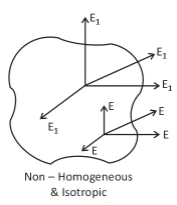Non-Homogenous and isotropic material

• Anisotropic Material
When a material exhibits different elastic properties at every direction at a every point than the material is known as Isotropic Material.
• Orthotropic Material
When a material exhibits Same elastic properties at only orthogonal direction at a given point than the material is known as Orthotropic Material.
For a homogeneous and isotropic material, the number of independent elastic constants are two.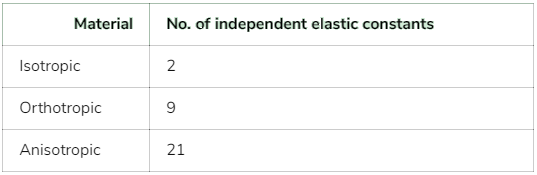Modules of Elasticity

When an axial load, P is applied along the longitudinal axis of a bar due to which length of the bar will be increases in the direction of applied load and a stress, σ is induced in the bar.
The ratio of stress to longitudinal strain, within elastic limits, is called the modulus of elasticity (E):

Thus, modules of Elasticity E = σ/ε

Poisson's Ratio

It is the ratio of lateral strain to the longitudinal strain.
It is an unitless quantity which is generally denoted as μ or 1/m.

Poisson's Ratio = - lateral strain/longitudinal strain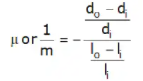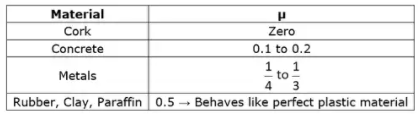Volumetric Strain

1. Volumetric Strain Due to Three Mutually Perpendicular Stress
Figure shows a parallelepiped subjected to three tensile load P1, P2 and P3 in the three mutually perpendicular direction.
Then,
δV / V = ε1 + ε2 + ε3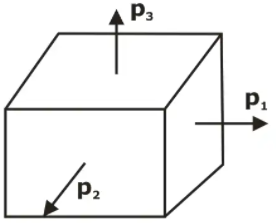Parallelepiped subjected to Three Mutually Perpendicular StressSince any axial load produces a strain in its own direction and an opposite kind of strain in every direction at right angles to this direction.
we have,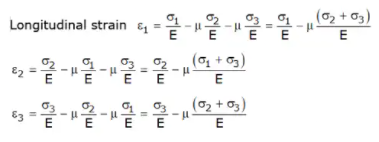Adding the three expressions of Equations we get,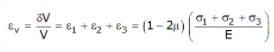2. Hydrostatic static state of stress
In case of hydrostatic state of stress, the applied stress in all direction are equal and tensile in nature.
i.e. σ1 = σ2 = σ3 = σ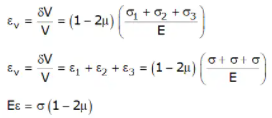since Eϵ and σ in the above expression are positive numbers, must also be positive.
1 - 2μ ≥ 0
2μ ≤ 1
μ ≤ 0.5
Thus, maximum value poison’s ratio is 0.5

3. Volumetric Strain Due to Single Direct Stress

Figure shows a rectangular bar of length L, width b and thickness t subjected to single direct load (P) acting along its longitudinal axis. Let this stress σ generated to be tensile in nature.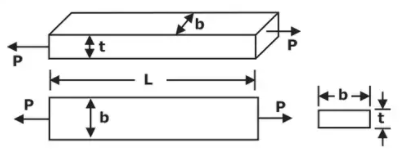Volumetric strain

Shear Modules or Modulus of Rigidity

The shear modules or modulus of rigidity expresses the relation between shear stress and shear strain.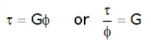where G = modulus of rigidity
ɸ = Shear strain (in radians) (also sometimes denoted by the symbol γ)

Bulk Modules

When a body is subjected to three mutually perpendicular like stresses of equal intensity (σ).
Then the ratio of direct stress (σ) to the corresponding volumetric strain (ϵv) is defined as the bulk modulus K for the material of the body.
Which is generally denoted as ‘K’

Thus K = Direct stress / Volumetric strain = σ / εv

Relation Between Different Elastic Properties

E = 2G(1 + μ)
E = 3K (1 - 2μ)
E = 9KG/3K + G

Value of any Elastic constant should be ≥ 0

E, K, G > 0
µ ≥ 0 [µcork = 0]
If K should be positive,
Then 1 – 2µ ≥ 0
μ ≤ 1/2
For any Engineering Material
0 ≤ µ ≤ 1/2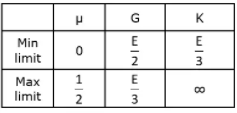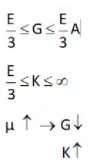Always
G ≤ E
For metals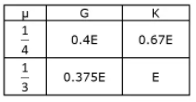Offer running on EduRev: Apply code STAYHOME200 to get INR 200 off on our premium plan EduRev Infinity!

## Strength of Materials (SOM)

35 videos|33 docs|48 tests

,

,

,

,

,

,

,

,

,

,

,

,

,

,

,

,

,

,

,

,

,

;